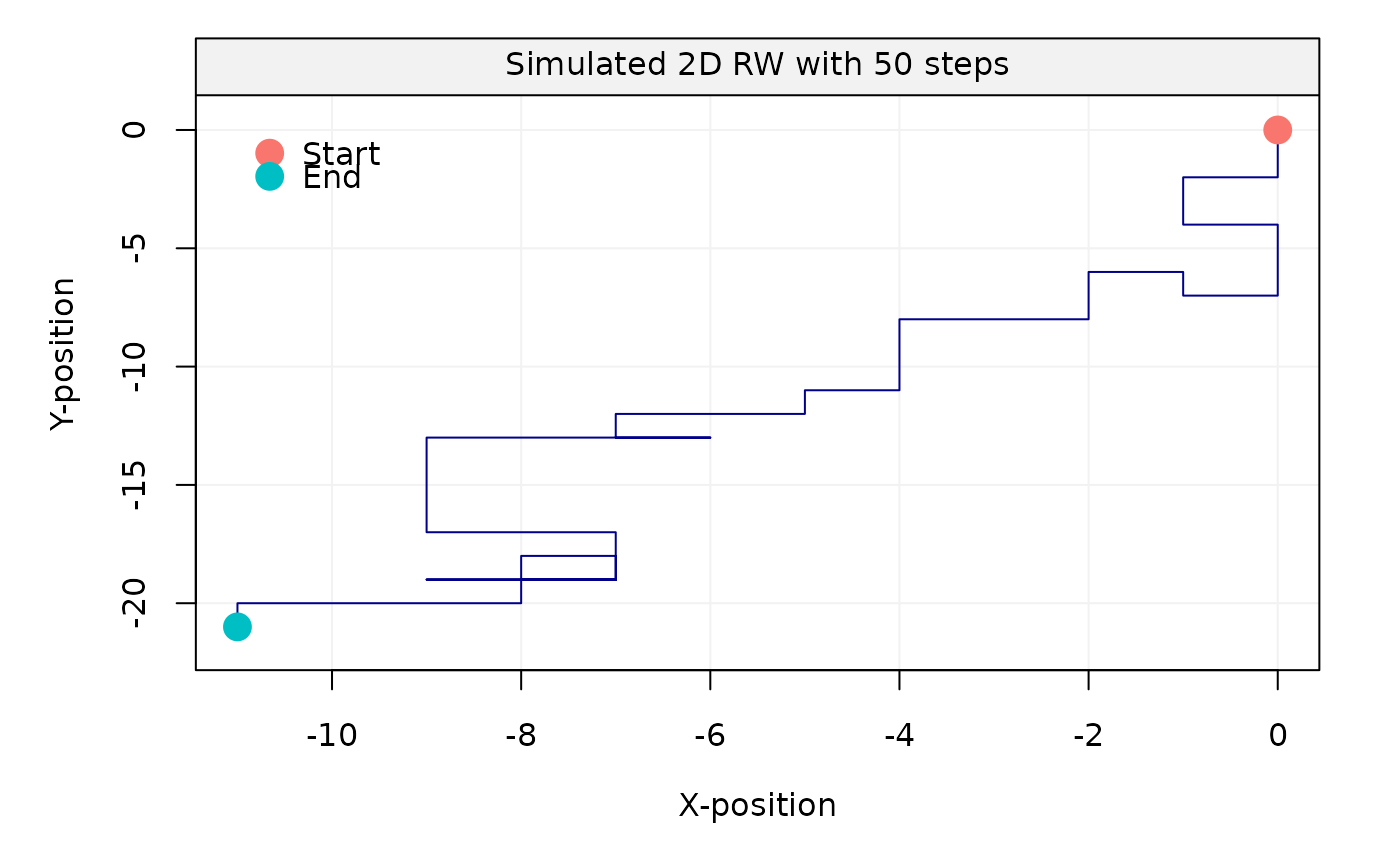The RW2dimension function computes direction random walk moves.

RW2dimension(steps = 100, probs = c(0.25, 0.5, 0.75))

## Arguments

steps

An integer that counts the number of steps of the random walk.

probs

A vector of double that specifies the probabilities to choose each direction.

## Author

Stéphane Guerrier

## Examples

RW2dimension(steps = 50, probs = c(0.2, 0.5, 0.6))